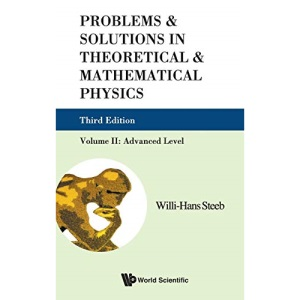30 Day Refund Guarantee# PROBLEMS AND SOLUTIONS IN THEORETICAL AND MATHEMATICAL PHYSICS, VOL II: ADVANCED LEVEL (3RD EDITION): 2

by STEEBWILLI-HANS (Author)

##### Synopsis

This book provides a comprehensive collection of problems together with their detailed solutions in the field of Theoretical and Mathematical Physics. All modern fields in Theoretical and Mathematical Physics are covered. It is the only book which covers all the new techniques and methods in theoretical and mathematical physics.Third edition updated with: Exercises in: Hilbert space theory, Lie groups, Matrix-valued differential forms, Bose-Fermi operators and string theory. All other chapters have been updated with new problems and materials. Most chapters contain an introduction to the subject discussed in the text.

### \$59.45

Save:\$0.70 (1%)

Quantity

10 in stock